# 物质复空间理论Mass Complex Space Theory

DOI: 10.12677/MP.2019.93016, PDF, HTML, XML, 下载: 640  浏览: 2,459

Abstract: Modern natural sciences are based on the postulations stepped from ancient Greek. Once the foundation is not solid or complete, the natural sciences will inevitably fall into various paradoxes and contradictions. Neglecting the guiding role of philosophical speculation in natural sciences, the mansion of natural sciences built and decorated in past four hundred years is very fragile despite its magnificent appearance. The law of unity of opposites, as the ultimate law of the universe, not only provides core world views but also core methodologies for human beings. Based on the achievements and contradictions of modern natural sciences and the Eastern classical philosophy, Mass Complex Space Theory (MCST) is the research and application of physical and mathematical rules created by the law of unity of opposites. MCST is compatible with the concept of space-time curvature of General Relativity and the wave-particle duality of Quantum Mechanics, it systemati-cally answered and resolved some critical problems and contradictions in physics.

1. 研究背景

1) 通过计算光在地表雷达站和月球表面放置的反射镜的往返时间，可以使地月距离的测量精确到毫米精度。月球激光测距表明：月球正以每年3.8厘米(1.5英寸)的平均速度螺旋远离地球  。

2) 通过接收从太阳系各天体返回的雷达波和跟踪星际旅行器的飞行，已经可以很准确地测定日地之间的距离。当前测得的最佳值是149,597,870.696 km。掌握了这种准确测度技术之后，俄国动力学家Gregoriy A. Krasinsky和Victor A. Brumberg在2004年测出地球正在逐渐远离太阳。虽然远离速度并不惊人——仅仅是每年15 cm，但这已经是测量误差的100多倍，足见有某种力量正在推动地球远离太阳  。

3) 来自先驱者10/11号、伽利略号和尤利西斯号宇宙飞船的无线电测量数据表明，作用在宇宙飞船上的加速度明显异常和恒定，大小为8.5 × 10−8 cm/s2，朝向太阳。两个独立的代码和物理策略被用来分析数据。已经排除了一些潜在的原因。我们讨论了未来的运动测试和可能的信号来源  。

4) 基于Robertson-McVittie度量，我们重新检验了宇宙膨胀是否导致Krasinsky和Brumberg (2004)报告的天文单位(长度)的增加。我们研究了宇宙学膨胀对测试粒子在这个时空中运动的影响。我们发现宇宙学的膨胀影响了这三个方面，但它们都微不足道。因此，我们确认宇宙学膨胀并不能解释观测到的DAU/dt  。

2. 物理学基础的批判

$gS=\left(GM/{R}^{2}\right)\ast 4\text{π}{R}^{2}=4\text{π}GM$

1) 认为引力场是由质体喷射出的以太形成，不能解释万有引力现象和质体的以太来源问题；

2) 认为引力场以太是静止的或均匀弥散的；

3) 把质体和引力场看作三维空间中并存的本体，即质能与场能并存；

4) 没能深刻地认识到空间的引力场化必然会导致空间的有限化；

5) 止步于数学中形而上学的复空间，没能对引力场空间进行物质意义上的复解析；

6) 误认为引力场传播的速度是光速，混淆了引力场速度和引力波速度的区别与联系。

3. 物质复空间理论的基础与假设

1) 数学中经典的复平面空间如何拓展为物质复空间；

2) 为兼容广义相对论，物质复空间可导出四维时空的弯曲机制；

3) 为兼容量子力学，物质复空间要具有量子化机制；

4) 为解决宇宙内部与外部的矛盾，物质复空间须具有闭合的互补结构；

5) 为解决物质与反物质的矛盾，物质复空间须具有反对称的互补结构；

6) 为解决第一推动的难题，物质复空间须具有內禀的动力学机制。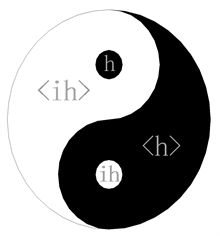Figure 1. Double singulars of conjugate complex space of quanta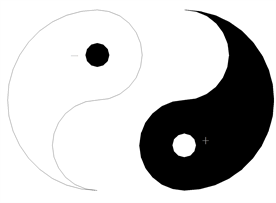Figure 2. Breaking of complex space of quanta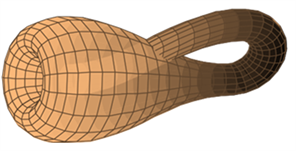Figure 3. Klein (bottle) complex space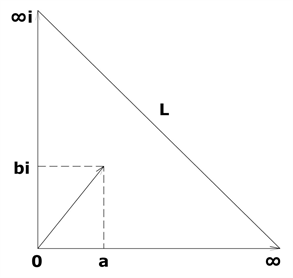Figure 4. Expanded complex plane

${\int }_{\infty }W\text{d}\tau =1$

$W={\psi }^{*}\psi$ 中， ${\psi }^{*}$ 为粒子的虚态即实空间(引力场)， $\psi$ 为粒子的实态即虚空间(斥力场)。

$\begin{array}{l}hv\left(客观形式\right)⇔H×iH\left(客体形态\right)\\ T⇔\frac{1}{iH}\\ {T}_{\mathrm{max}}⇔\frac{1}{ih}\end{array}$

4. 物质复空间理论的公设

MCST第一公设：物质是由对立统一的虚实空间相互作用生成。

MCST第二公设：实空间是收敛的引力场，虚空间是发散的斥力场。

MCST第三公设：物质是超循环物质态的局域耦合与分化的表象(图5)。

MCST第四公设：超循环物质态的运动遵循物极必反的自然法则。

MCST第五公设：实空间是物质的虚态，虚空间是物质的实态。

MCST第六公设：多质体引力场流的分布遵守最小作用原理。

MCST第七公设：质体的运动是其引力场空间外在不平衡的效应。

MCST第八公设：在物质复空间的临界域，引力场流速等于引力场波速。

MCST第九公设：物质复空间场流的分布具有力学惯性。

MCST第十公设：质体本征复空间的临界域截面与其本征质量成正比。

MCST第一定理：宇宙的极大尺寸与极小尺度是对立统一。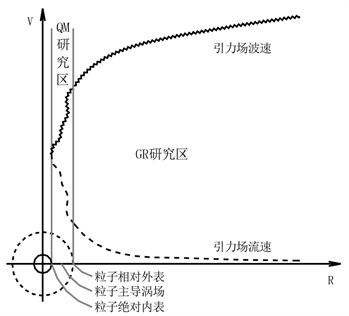Figure 5. Flow velocity and wave velocity of a gravitation field

MCST第二定理：相互靠近的一对粒子，二者复空间的临界域均增大。

5. 物质复空间理论的场论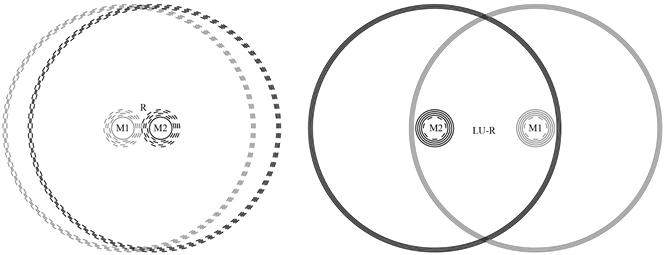Figure 6. Interactions between two mass bodies

$\begin{array}{l}F\left(R\right)=G{M}_{1}{M}_{2}/{R}^{2}+iG{M}_{1}{M}_{2}/{\left(lU-R\right)}^{2}\\ \text{\hspace{0.17em}}\text{\hspace{0.17em}}\text{\hspace{0.17em}}\text{\hspace{0.17em}}\text{\hspace{0.17em}}\text{\hspace{0.17em}}\text{\hspace{0.17em}}\text{\hspace{0.17em}}\text{ }=G{M}_{1}{M}_{2}\left[1/{R}^{2}+i/{\left(lU-R\right)}^{2}\right]\\ |F|=G{M}_{1}{M}_{2}\sqrt{{\left(1/{R}^{2}\right)}^{2}+{\left[1/{\left(lU-R\right)}^{2}\right]}^{2}}\end{array}$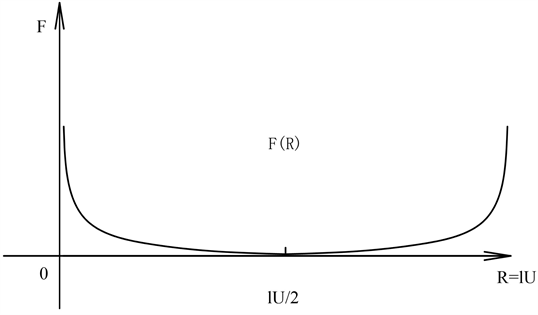Figure 7. Gravitational force distribution between two mass bodies

1) 质体成形于其自身复空间的临界域；

2) 质体是临界复空间引力场流涡旋和共轭的表象；

3) 质体复空间临界域的球形截面大小与其本征质量正比；

4) 多质体系统中，所有质体的临界域均自发扩张，形成引力质量应力；

5) 引力作用的物理机制可类比于现实流体的场流分布，并可通过流体实验模拟出来；

6) 质体的引力质量恒等于其惯性质量(临界域的空间流入恒等于其空间流出)；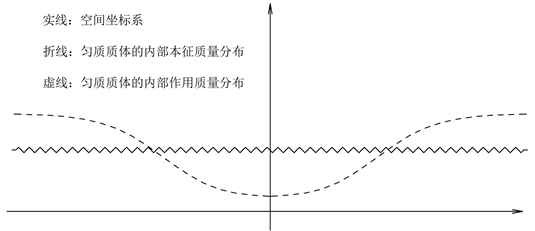Figure 8. Flow distribution of gravitation field of homogeneous mass

7) 重力秒加速度是引力场空间的净流速(运动是由质体的空间结构决定的)；

8) 引力场的流速小于或等于引力场的波速(目的是引入复空间的临界域)；

9) 引力场的波速与真空密度成反比(类比于现实流体)。

${C}_{m}{H}_{m}={{C}^{\prime }}_{m}{{H}^{\prime }}_{m}$

${{C}^{\prime }}_{m}={C}_{m}{H}_{m}/{{H}^{\prime }}_{m}$

$Gm/{r}_{0}^{2}={C}_{m}{s}^{-1}$

$Gm/{{r}^{\prime }}_{0}^{2}={{C}^{\prime }}_{m}{s}^{-1}$

${{r}^{\prime }}_{0}=\sqrt{\frac{Gms}{{{C}^{\prime }}_{m}}}$

$\Delta {r}_{0}=\sqrt{\frac{Gms}{{{C}^{\prime }}_{m}}}-\sqrt{\frac{Gms}{{C}_{m}}}$

$\Delta {S}_{m}=4\text{π}{{r}^{\prime }}_{0}^{2}-4\text{π}{r}_{0}^{2}$

${V}_{R}=GMs/{R}^{2}$${S}_{R}=4\text{π}{R}^{2}$

$\Delta M=\frac{\Delta {S}_{m}}{{S}_{R}}M=\frac{4\text{π}{{r}^{\prime }}_{0}^{2}-4\text{π}{r}_{0}^{2}}{4\text{π}{R}^{2}}M=\frac{{{r}^{\prime }}_{0}^{2}-{r}_{0}^{2}}{{R}^{2}}M$

${{C}^{\prime }}_{m}={C}_{m}{H}_{m}/{{H}^{\prime }}_{m}={C}_{m}{H}_{m}/\left({H}_{m}+\frac{GM}{{R}^{2}}\right)$

${{r}^{\prime }}_{0}=\sqrt{\frac{Gms}{{{C}^{\prime }}_{m}}}=\sqrt{\frac{Gms}{{C}_{m}{H}_{m}/\left({H}_{m}+\frac{GM}{{R}^{2}}\right)}}$

$\Delta M=\frac{{{r}^{\prime }}_{0}^{2}-{r}_{0}^{2}}{{R}^{2}}M=\frac{\frac{Gms}{{C}_{m}{H}_{m}/\left({H}_{m}+\frac{GM}{{R}^{2}}\right)}-\frac{Gms}{{C}_{m}}}{{R}^{2}}M=\frac{GMms}{{R}^{2}}\ast \frac{\frac{GM}{{R}^{2}}}{{C}_{m}{H}_{m}}$

$⇒\frac{\Delta m}{\Delta M}=\frac{\frac{GmMs}{{R}^{2}}\ast \frac{\frac{Gm}{{R}^{2}}}{{C}_{M}{H}_{M}}}{\frac{GMms}{{R}^{2}}\ast \frac{\frac{GM}{{R}^{2}}}{{C}_{m}{H}_{m}}}=\frac{m{C}_{m}{H}_{m}}{M{C}_{M}{H}_{M}}=\frac{m}{M}$

MCST第三定理：距离其它质体足够远的一对质体，各自截获对方的引力质量与二者的本征质量成反比。

$GQ/{R}_{Q}^{2}={H}_{0}$

${m}^{\prime }=m+\frac{{H}_{0}^{2}ms}{{C}_{m}{H}_{m}}$

$\Delta {Q}_{M}=\frac{GQMs}{{R}_{M}^{2}}\ast \frac{\frac{GQ}{{R}_{M}^{2}}}{{C}_{M}{H}_{M}}=\frac{{H}_{0}^{2}Ms}{{C}_{M}{H}_{M}}$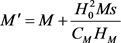${C}_{m}{H}_{m}={C}_{M}{H}_{M}=w$，w为真空光速常数(w与精细结构常数等效)

${m}^{\prime }=m\left(1+\frac{{H}_{0}^{2}}{w{s}^{-1}}\right)$

${M}^{\prime }=M\left(1+\frac{{H}_{0}^{2}}{w{s}^{-1}}\right)$

$⇒\frac{{m}^{\prime }}{{M}^{\prime }}=\frac{m}{M}$

MCST第四定理：质体从背景真空中截获的引力质量与质体的本征质量成正比。

MCST第五定理：质体的引力质量恒等于其惯性质量。

(因临界空间实部的引力场流入量恒等于其虚部的斥力场流出量)

MCST第六定理：对于近距离作用的一对质体，质体在实空间截获的引力质量，在虚空间全部返还给与其作用的质体。

(因虚实空间反对称，实空间的近距离在虚空间表象为远距离)

MCST第七定理：稳态的天体系统，中心天体的引力作用质量总是小于其本征质量的，外围天体的引力作用质量总是大于其本征质量的。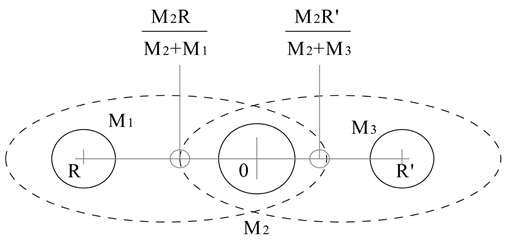Figure 9. Gravitational interactions among three mass bodies

M1与M2组合体的左重心到中心天体M2的距离为： $\frac{{M}_{2}R}{{M}_{2}+{M}_{1}}$

M2与M3组合体的右重心到中心天体M2的距离为： $\frac{{M}_{2}R}{{M}_{2}+{M}_{3}}$

M1截获的引力质量： $\frac{K\left({M}_{2}+{M}_{3}\right)}{{\left(R+\frac{{M}_{2}{R}^{\prime }}{{M}_{2}+{M}_{3}}\right)}^{2}}$

M1损失的引力质量： $\frac{K{M}_{1}}{{\left(R+\frac{{M}_{2}{R}^{\prime }}{{M}_{2}+{M}_{3}}\right)}^{2}}$

M1的作用质量：

${M}_{1}+\frac{K\left({M}_{2}+{M}_{3}\right)}{{\left(R+\frac{{M}_{2}{R}^{\prime }}{{M}_{2}+{M}_{3}}\right)}^{2}}-\frac{K{M}_{1}}{{\left(R+\frac{{M}_{2}{R}^{\prime }}{{M}_{2}+{M}_{3}}\right)}^{2}}={M}_{1}+\frac{K\left({M}_{2}+{M}_{3}-{M}_{1}\right)}{{\left(R+\frac{{M}_{2}{R}^{\prime }}{{M}_{2}+{M}_{3}}\right)}^{2}}$

M2的作用质量： ${M}_{2}-\frac{K\left({M}_{2}+{M}_{3}-{M}_{1}\right)}{{\left(R+\frac{{M}_{2}{R}^{\prime }}{{M}_{2}+{M}_{3}}\right)}^{2}}-\frac{K\left({M}_{2}+{M}_{1}-{M}_{3}\right)}{{\left({R}^{\prime }+\frac{{M}_{2}R}{{M}_{2}+{M}_{1}}\right)}^{2}}$

${\left(\epsilon \mu \right)}^{\wedge -1/2}\sum |{g}_{i}|=w$

6. 太阳系背景真空相对密度的计算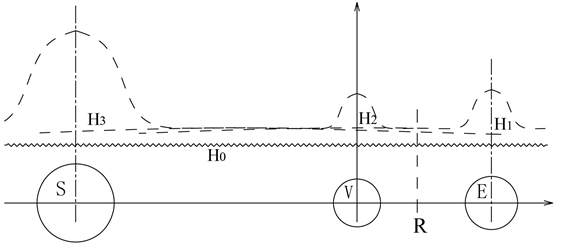Figure 10. Flow distribution of gravitation field between the Earth and the Sun

$\begin{array}{l}{C}_{R}=\frac{{H}_{E}}{{H}_{R}}{C}_{E}=\frac{{H}_{E}{C}_{E}}{\frac{GM}{{\left({L}_{2}+R\right)}^{2}}+\frac{G{m}^{\prime }}{{R}^{2}}+\frac{Gm}{{\left({L}_{1}-R\right)}^{2}}+{H}_{0}}\\ {H}_{E}=\frac{GM}{{\left(L-{R}_{1}\right)}^{2}}+\frac{G{m}^{\prime }}{{\left({L}_{1}-{R}_{1}\right)}^{2}}+\frac{Gm}{{R}_{1}^{2}}+{H}_{0}\end{array}$

$GM/{H}_{E}{C}_{E}=a$$G{m}^{\prime }/{H}_{E}{C}_{E}=b$$Gm/{H}_{E}{C}_{E}=d$${H}_{0}/{H}_{E}{C}_{E}=f$

$\Delta t=\frac{\Delta R}{{C}_{R}}=\left[\frac{a}{{\left({L}_{2}+R\right)}^{2}}+\frac{b}{{R}^{2}}+\frac{d}{{\left({L}_{1}-R\right)}^{2}}+f\right]\Delta R$ $\Delta t$ 为真空光速时间离散值

$\int \text{d}t=\int \frac{a}{{\left({L}_{2}+R\right)}^{2}}+\frac{b}{{R}^{2}}+\frac{d}{{\left({L}_{1}-R\right)}^{2}}+f\text{d}R+Con.=\frac{-a}{{L}_{2}+R}-\frac{b}{R}+\frac{d}{{L}_{1}-R}+fR+Con.$

${\int }_{{R}_{2}}^{{L}_{1}-{R}_{1}}\text{d}t=a\left(\frac{1}{{L}_{2}+{R}_{2}}-\frac{1}{L-{R}_{1}}\right)+b\left(\frac{1}{{R}_{2}}-\frac{1}{{L}_{1}-{R}_{1}}\right)+d\left(\frac{1}{{R}_{1}}-\frac{1}{{L}_{1}-{R}_{2}}\right)+f\left({L}_{1}-{R}_{1}-{R}_{2}\right)=T$

T为实测的已知参数，根据地日几何距离和地金几何距离，可计算出太阳系背景真空相对密度。进而，我们可推算出太阳辐射到地球的理论时间，修正后的时间要小于过去根据真空光速不变计算的时间。

7. 真空相对密度的测量Figure 11. Curvature of space-time caused by mass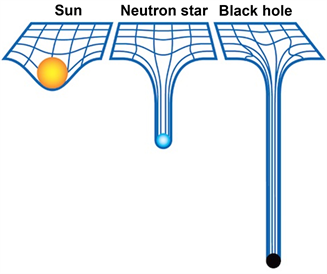Figure 12. Comparison of curvatures of space-time

1) 引力几何化的时空弯曲会导致临近引力场源的真空密度小于宇宙深空场的真空密度；

2) 引力几何化的平滑时空与量子力学所揭示的真空涨落相违背；

3) 静态的引力几何化不可能产生量子自旋态，导致了广义相对论和量子力学根本的矛盾与对立；

4) 引力几何化无法通过物理实验模拟出多质体引力场作用的复杂联络与二次分布规律。

1) 实验目的

a) 否定真空光速不变的假设

b) 验证物质复空间理论

c) 重建深空探测的理论基础

2) 真空相对密度测量的理论基础

3) 真空磁导率的测试方案

4) 真空磁导率测试的对照环境

5) 真空磁导率测试的注意事项

a) 地表模拟空间站与空间站的微气候环境须要高度相同

b) 使用同一套测量设备往返于太空和地表进行每天同时段的分时测量

6) 对测试结果的理论预测

$\left({g}_{R}+V\right)/\left({g}_{B}+V\right)={\mu }_{R}/{\mu }_{B}$

${\mu }_{B}={\mu }_{R}\left({g}_{B}+V\right)/\left({g}_{R}+V\right)$

8. 宇宙的结构与尺度

$\left({g}_{R}+V\right)/\left({g}_{B}+V\right)={\mu }_{R}/{\mu }_{B}$

$V=\left({g}_{R}{\mu }_{B}-{g}_{B}{\mu }_{R}\right)/\left({\mu }_{R}-{\mu }_{B}\right)$$\left({g}_{R}+V\right)C={C}_{h0}^{2}{s}^{-1}$

${C}_{h0}^{2}=s\left[{g}_{R}+V\right]C$

$a=G{m}_{0}/{R}^{2}=Gh{s}^{-1}/{\left(CR\right)}^{2}\le {C}_{h0}{s}^{-1}$${C}_{h0}$ 为量子h的临界波速

$Gh{s}^{-1}/{\left(C{R}_{0}\right)}^{2}={C}_{h0}{s}^{-1}$

${R}_{0}=\sqrt{\frac{Gh}{{C}^{2}{C}_{h0}}}$

${l}_{U}=1/{R}_{0}=1/\sqrt{\frac{Gh}{{C}^{2}{C}_{h0}}}$

${l}_{U}$ 为量子h的宇宙半径，量纲取反。考量到地表真空密度对量子复空间临界域的影响，地表的普朗克长度须要进行背景真空因素修正，地表背景真空修正后的普朗克长度会比根据相对论和量子力学给出的普朗克长度略大一些。自然的宇宙复空间，则是无数的复合量子 $h×ih$ 小宇宙复空间的动态集合体。

9. 物质复空间理论的应用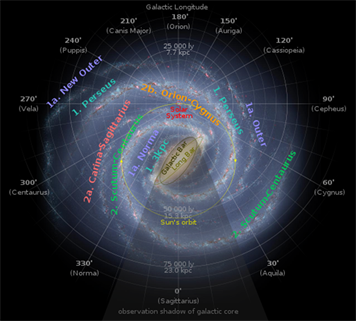Figure 13. Solar system steps into the zone of increasing density of vacuumTable 1. Comparison of the explanations for astronomical observation

10. 总结

  John D. Andeson, 文. Shea, 译. 未知的引力[DB/OL]. https://www.guokr.com/blog/257452, 2012-06-30.  Wikipedia. Lunar Distance (Astronomy). https://en.wikipedia.org/wiki/Lunar_distance_(astronomy)  钟翠相. 地球及其他行星远离太阳的真正原因和减缓全球气候变暖的一种对策[J]. 科技视界, 2016(25): 9-10. https://doi.org/10.12968/sece.2016.25.10a  Anderson, J.D., Laing, P.A., Lau, E.L., Liu, A.S., Nieto, M.M. and Turyshev, S.G. (1998) Indication, from Pioneer 10/11, Galileo, and Ulysses Data, of an Apparent Anomalous, Weak, Long-Range Acceleration. Physical Review Letters, 81, 2858. https://doi.org/10.1103/PhysRevLett.81.2858  Arakida, H. (2007) Does Astronomical Unit Increase?: Cosmological Expansion and Solar System Dynamics. https://www.researchgate.net/publication/289360540 _Does_astronomical_unit_increase_Cosmological_expansion_and_solar_system_dynamics  姜祖桐. H自组织理论[M]. 上海: 上海科学技术文学出版社, 2007: 278.  Baez, J. (2011) What’s the Energy Density of the Vacuum. http://math.ucr.edu/home/baez/vacuum.html  汤川秀树. 创造力与直觉[M]. 石家庄: 河北科技出版社, 2000: 142.  沈汇涛. 真空是真的空吗? [DB/OL]. https://wemedia.ifeng.com/62324441/wemedia.shtml, 2018-05-26.